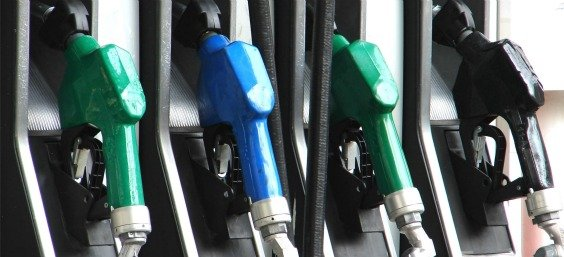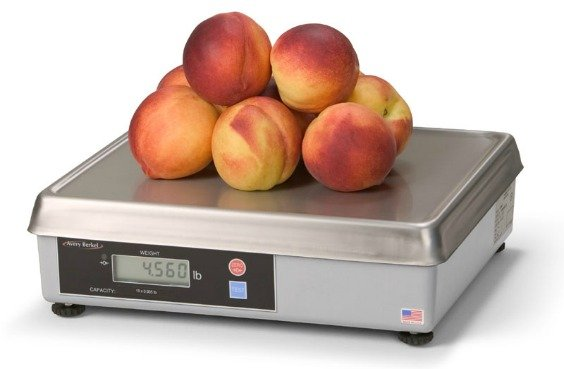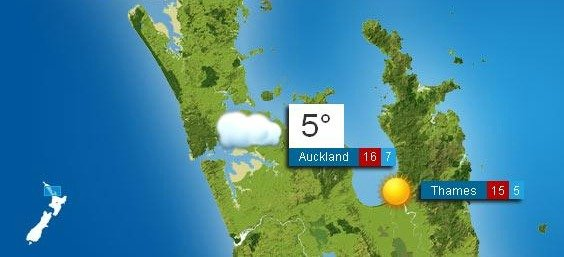##### A US Traveller’s Crash Course in Metric…

It’s time to wrap our heads around another fun transition to face as we travel on the journey…

The Metric System

The United States is one of only 3 remaining countries on the planet not yet using the system of measurement (we join the world powerhouses of Liberia and Myanmar in this somewhat sad distinction), so in prepping for days on the road, making our own meals, and basically trying to not stick out like a sore thumb, here is a short crash course in the metric system for anyone that maybe traveling internationally in the future.

Crash Course Topic 1:  Speed and Distance (US Miles vs. Metric Kilometers)Speed and distance are for the most part presented in Kilometers (kms) for distance or in Kilometers Per Hour (kph) for speed and speed limits.
The conversion is pretty straight forward: 1 kilometer = .6 of a mile
Doing the Math:
100 kms = 60 Miles or multiply the number of kilometers by .6 to get the US equivalent in miles.
Alternatively, you can multiply the number of kilometers by 3 and then divide the total by 5.  (Example:  200 kms * 3 = 600 /5 = 120 Miles)

Crash Course Topic 2:  Fuel & Other Liquids (US Gallons vs. Metric Liters)Fuel and other liquids use liters (kms) for measurement of fuels and other liquids.
This conversion is a bit more painful: 1 liter = .2641 of a gallon or 1 gallon = 3.785 liters
Doing the Math:
38 liters = Approx. 10 Gallons
At \$6.50 USD a gallon (\$2.10NZD per litre) for gas, you can be assured we’ll be paying close attention to our math here.

Crash Course Topic 3:  The Grocery Store & Food (US Pounds and Ounces vs. Metric Grams)Food weights are made in grams (g) and kilograms (kg) for most foods.
This conversion is not bad, but has a number of switchups:
1000 grams = 1 kilogram = 2.2 pounds = 35.3 ounces

Doing the Math:
Multiply the number of kilograms by 2.2 to get # of pounds
Use the following guides for weights in grocery store or market:
100 grams = 3 1/2 ounces
450 grams = Approx. 1 pound

Crash Course Topic 4:  Weather (US Fahrenheit vs. Metric Celsius)Temperature is measured in degrees Celsius (C)
This conversion is straightforward:
0 degrees Celsius = 32 degrees Fahrenheit
For every degree Celsius increase, add 1.8 degrees Fahrenheit
(as long as you stay above 0 degrees Celsius = 32 degrees Fahrenheit)

Doing the Math:
Multiply the number of degrees Celsius by 1.8 and then add 32 to get a Fahrenheit temperature.
Use the following guides as starting points:
0 Celsius = 32 Fahrenheit
10 Celsius = 50 Fahrenheit
20 Celsius = 68 Fahrenheit
37 Celsius = 98.6 Fahrenheit (Normal Body Temperature)
150 Celsius = Approx. 300 Fahrenheit (For Cooking)
200 Celsius = Approx. 400 Fahrenheit (For Cooking)
250 Celsius = Approx. 500 Fahrenheit (For Cooking)

We will undoubtedly hit a few snags along the way, but think that the above information will give us a good start now that we’re here!